# Beckman-Quarles-type theorems

(diff) ← Older revision | Latest revision (diff) | Newer revision → (diff)

A fundamental theorem in Euclidean geometry is the following result of F.S. Beckman and D.A. Quarles [a1]. Letbe a fixed positive real number and let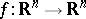() be a mapping satisfying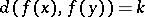for allwith. (Here,denotes the Euclidean distance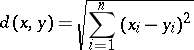of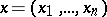and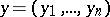in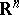.) The mappingis then in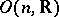.

It should be emphasized that no regularity assumption (like differentiability or continuity) is required in the theorem. For an analogue in hyperbolic geometry, see [a4] and [a5].

A distance space is a set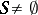, a set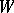and a mapping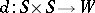. The element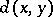is called the distance ofand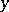(in this order). Beckman–Quarles-type theorems for distance spaces are, up to generalizations, statements claiming that a mapping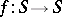preserves all occurring distancesif it preserves one single distance. Beckman–Quarles-type theorems belong to a class of statements called characterizations of geometrical mappings under mild hypotheses.

Let, for. Let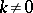be a fixed real number and let() be a mapping satisfying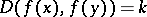for allwith. Thenis a Lorentz transformation of. Forand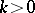, this was proved by J. Lester; forand for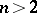andthis was proved by W. Benz (see [a2] for all these results). The proofs are different for the three cases, and no common proof is known (1996).

Letandbe normed real vector spaces such thatis strictly convex and the dimension ofis at least(cf. also Vector space; Convex set). Letbe a fixed real number and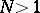a fixed integer. Suppose thatis a mapping satisfying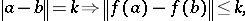for all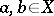. Thenis an isometric operator, and hence an affine transformation (the Benz–Berens theorem, [a2]). Ifand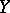are, in addition, pre-Hilbert spaces (cf. Pre-Hilbert space), then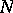may be replaced by any real number greater than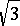(Radó–Andreascu–Valcán theorem).

There are generalizations for finite planes [a9], rational or constructible curves (B. Farrahi), non-Euclidean spaces [a11], and planes over fields [a10]. F. Radó [a8] has proved the following theorem. Letbe a non-singular metric vector space of dimension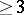over,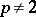,, and letbe a fixed element of. Ifis a bijection ofpreserving distance, thenis a semi-affine mapping if.

Let,, be a mapping satisfying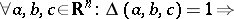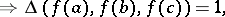where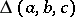denotes the area of the triangle with vertices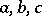. Thenis a Euclidean motion, i.e.,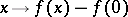is in(Lester's theorem), [a3]. Forthe equi-affine mappings are characterized similarly.

Letbe a mapping of the set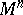of lines in,, into itself such that whenever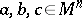are the lines making up the sides of a triangle of area, then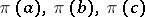are also the sides of a triangle of area. Thenis induced by a Euclidean motion offorand by an equi-affine mapping if(the Wen-ling Huang theorem, [a3]).

Letbe a fixed real number anda normed real vector space of dimension. Let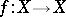be a function satisfying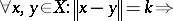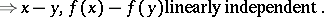Then there are elements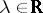and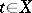such that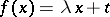for all. This theorem was proved by Benz [a2] and, anew, by D. Laugwitz [a6].

General references for this area are [a2], [a3] and [a7].

How to Cite This Entry:
Beckman-Quarles-type theorems. Encyclopedia of Mathematics. URL: http://encyclopediaofmath.org/index.php?title=Beckman-Quarles-type_theorems&oldid=19099
This article was adapted from an original article by W. Benz (originator), which appeared in Encyclopedia of Mathematics - ISBN 1402006098. See original article